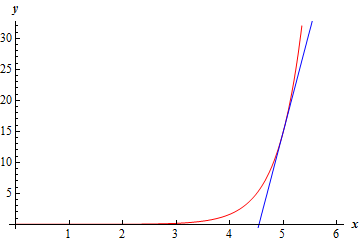Paul's Online Notes
Home / Calculus I / Applications of Derivatives / Linear Approximations
Show Mobile Notice Show All Notes Hide All Notes
Mobile Notice
You appear to be on a device with a "narrow" screen width (i.e. you are probably on a mobile phone). Due to the nature of the mathematics on this site it is best views in landscape mode. If your device is not in landscape mode many of the equations will run off the side of your device (should be able to scroll to see them) and some of the menu items will be cut off due to the narrow screen width.

### Section 4.11 : Linear Approximations

1. Find a linear approximation to $$f\left( x \right) = 3x\,{{\bf{e}}^{2x - 10}}$$ at $$x = 5$$.

Show All Steps Hide All Steps

Start Solution

We’ll need the derivative first as well as a couple of function evaluations.

$f'\left( x \right) = 3{{\bf{e}}^{2x - 10}} + 6x\,{{\bf{e}}^{2x - 10}}\hspace{0.5in}f\left( 5 \right) = 15\hspace{0.5in}f'\left( 5 \right) = 33$ Show Step 2

There really isn’t much to do at this point other than write down the linear approximation.

$\require{bbox} \bbox[2pt,border:1px solid black]{{L\left( x \right) = 15 + 33\left( {x - 5} \right) = 33x - 150}}$

While it wasn’t asked for, here is a quick sketch of the function and the linear approximation.